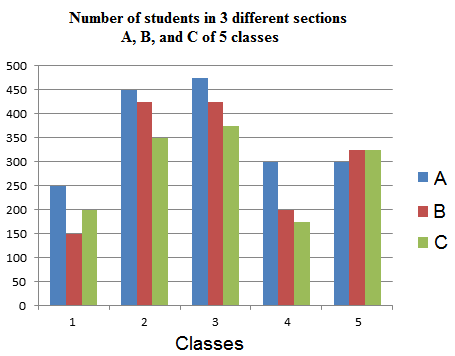## Data Interpretation for SBI PO 2017

Directions (1 – 5): Study the table carefully to answer the questions that follow:-
Course – wise number of students in different cities

 Course city MFC MIB MCA MBM MPM Ahmadabad 1234 1384 1440 1289 1332 Bengaluru 1156 1783 1874 1003 1340 Bhopal 1187 1347 1532 1532 1486 Chennai 1342 1473 1129 1129 1666 New Delhi 1230 1098 118 1128 1777 Hyderabad 1456 1234 1556 1556 1789 Kolkata 1239 1785 1865 1865 1762
1. In Chennai, the number of MFC students is approximately what percent of that of MPM students?
a) 69
b) 90
c) 94
d) 73
e) 81
2. In Ahmedabad, the number of MBM students is approximately what percent of that of MCA students?
a) 66
b) 71
c) 84
d) 87
e) 90
3. What is the difference between total number of MIB and MFC students from all the cities together?
a) 1140
b) 1260
c) 1330
d) 1480
e) None of these
4. Which City has the highest number of students?
b) Bengaluru
c) Chennai
d) Kolkata
e) None of these
5. What is the approximate average number of students enrolled in MIB course from all the cities together?
a) 1263
b) 1165
c) 1443
d) 1503
e) 1349
Directions (6-10): Study the following bar graph and answer the following questions.6. What is the total number of children who are in section B in all the classes together?
a) 1775
b) 1625
c) 1595
d) 1535
e) 1525
7. What is the respective ratio of the total number of students in sections A, B and C in all the classes together?
a) 24 : 21 : 19
b) 18 : 17 : 16
c) 71 : 61 : 57
d) 48 : 42 : 37
e) none of these
8. Number of students of section B in class 3 forms approximately what percent of the total number of students of section B from all the classes together?
a) 24 %
b) 18%
c) 41%
d) 38%
e) 28%
9. Number of students of section A in class 1 forms what percent of total number of students in class 1 together?
a) 41.67%
b) 44.16%
c) 55.24%
d) 46.64%
e) none of these
10. Total number of students in class 2 is how much % less than in classes 4 and 5 together?
a) 24.62%
b) 28.43%
c) 26.76%
d) 20.50%
e) none of these
Solutions:
1. E) Number of students of MFC in Chennai = 1342
Number of students of MPM in Chennai = 1666
Required percentage = 1342/1666 x 100 = 80.55 = 81
2. E) Number of MBM students in Ahmedabad = 1289
Number of MCA students in Ahmedabad = 1440
Required percentage = 1289/1440 x 100 = 89.5 = 90
3. B) Total Number of MIB  students in all citites = 10104
Total Number of MFC students in all cities = 8844
Difference 10104 – 8844 = 1260
4. D) Total number of students :
Bengaluru – 1156 + 1783 + 1874 + 1003 + 1340 = 7156
Chennai – 1342 + 1473 + 1129 + 1765 + 1666 = 7375
New Delhi – 1230 + 1098 + 1128 + 1865 + 1777 = 7098
Hyderabad – 1456 + 1234 + 1556 + 1653 + 1789 = 7688
Kolkata – 1239 + 1785 + 1865 + 1504 + 1762 = 8155
The number of students was highest in Kolkata.
5. C) Number of students enrolled in MIB course = 1384 + 1783 + 1347 + 1473 + 1098 = 10104
Required average = 10104/7 = 1443
6. E) Total number of children who are in section B in all the classes together = 150+425+425+200+325 = 1525
7. C) Total number of students in Section A: 1775, Section B: 1525 and Section C: 1425
Therefore required ratio = 1775:1525:1425 = 71 : 61 : 57
8. E) Section B, class 3 = 425
Total in section B = 1525
So required % = 425/1525 * 100 = 28%
9. A) Section A, class 1 = 250
Total in class 1 = 250+150+200 = 600
So required % = 250/600 * 100 = 41.67%
10. A) Class 2 = 450+425+350 = 1225
Class 4 + 5 = 300+200+175 + 300+325+325 = 1625
So required % = (1625-1225)/1625 * 100 = 24.62%